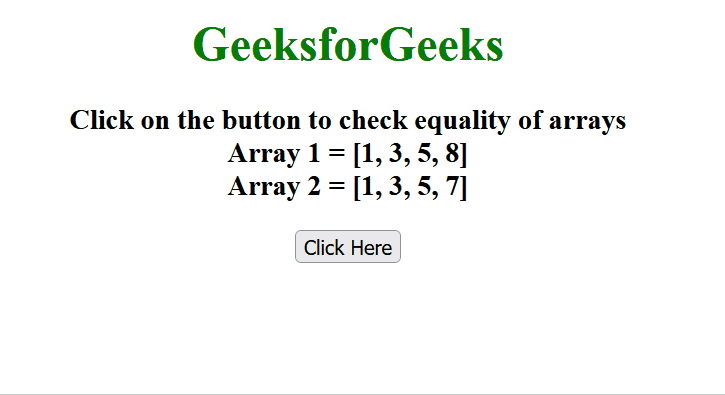Open In App

# How to compare two JavaScript array objects using jQuery/JavaScript ?

In this article, we are given two JavaScript array/array objects and the task is to compare the equality of both array objects.

These are the methods to compare two JavaScript array objects:

## Approach 1: Using jQuery not() method

• Use the jQuery not() method to check for each element of array1, if it is not present in array2 or for each element of array2, if this is not present in array1, then it returns false in both cases.
• Also, check the length of both arrays.

Example: This example uses the jQuery not() method to compare the equality of both arrays.

## html

 ```<``html` `lang``=``"en"``>` `<``head``>``    ``<``script` `src``=``"https://ajax.googleapis.com/ajax/libs/jquery/3.4.1/jquery.min.js"``>``    ``` `    ``<``title``>``        ``How to compare two JavaScript array``        ``objects using jQuery/JavaScript ?``    ````` `<``body` `style``=``"text-align:center;"``>``    ``<``h1` `style``=``"color:green;"``>``        ``GeeksforGeeks``    ``` `    ``<``h3``>``        ``Click on the button to check``        ``equality of arrays<``br``>``        ``Array 1 = [1, 3, 5, 8]<``br``>``        ``Array 2 = [1, 3, 5, 7]``    ``` `    ``<``button` `onclick``=``"GFG_Fun()"``>``        ``Click Here``    ``` `    ``<``h3` `id``=``"GFG"``        ``style``=``"color: green;"``>``    ``` `    ``<``script``>``        ``let elm = document.getElementById('GFG');``        ``let arr1 = [1, 3, 5, 8];``        ``let arr2 = [1, 3, 5, 7];` `        ``function GFG_Fun() {``            ``elm.innerHTML =``                ``\$(arr1).not(arr2).length === 0 &&``                ``\$(arr2).not(arr1).length === 0;``        ``}``    ````` ``

Output:## Approach 2: Use the sort() function

• First, use the sort() function to sort both arrays in ascending order.
• Then start matching the element one by one and if there is any mismatch found then it returns false otherwise it returns true.

Example: This example uses JavaScript`s sort() method to sort the array in ascending order then it checks for the same values.

## Javascript

 `let arr1 = [1, 3, 7, 5];``let arr2 = [1, 3, 5, 7];` `function` `compare(ar1, ar2) {``    ``ar1.sort();``    ``ar2.sort();` `    ``if` `(ar1.length != ar2.length)``        ``return` `false``;` `    ``for` `(let i = 0; i < ar1.length; i++) {``        ``if` `(ar1[i] != ar2[i])``            ``return` `false``;``    ``}``    ``return` `true``;``}` `console.log(compare(arr1, arr2));`

Output

```true

```

## Approach 3:JSON.stringify() function

• Create two array objects and store them into arr1 and arr2 variables.
• Use JSON.stringify() function to convert an object into a JSON string.
• Now compare both JSON strings using the comparison operator (==) to check whether both array objects are equal or not.

Note: This method works only when both array objects are sorted in the same fashion.

Example: In this example, we will be using JSON.stringify() function to compare two array objects.

## Javascript

 `let arr1 = [``    ``{ id: ``"1"``, name: ``"GeeksforGeeks"` `},``    ``{ id: ``"2"``, name: ``"GFG"` `}``];` `let arr2 = [``    ``{ id: ``"1"``, name: ``"GeeksforGeeks"` `},``    ``{ id: ``"2"``, name: ``"GFG"` `}``];` `function` `compare(ar1, ar2) {``    ``if` `(JSON.stringify(ar1) == JSON.stringify(ar2)) {``        ``return` `true``;``    ``}``    ``else``        ``return` `false``;``}` `console.log(compare(arr1, arr2));`

Output

```true

```

## Approach 4: Using every() and indexOf()

We can use every() to iterate through every array element. The JavaScript Array indexOf() Method is used to find the index of the first occurrence of the search element provided as the argument to the method.

Example:

## Javascript

 `let array1 = [1, 2, 3];``let array2 = [1, 2, 3];` `let isEqual = array1.length === array2.length &&``    ``array1.every(``function` `(element, index) {``        ``return` `element === array2[index];``    ``});` `console.log(isEqual);`

Output

```true

```

## Approach 5: Using Lodash _.isEqual() Method

The Lodash _.isEqual() Method performs a deep comparison between two values to determine if they are equivalent.

Example:

## Javascript

 `// Defining Lodash variable``const _ = require(``'lodash'``);` `let val1 = { ``"a"``: ``"gfg"` `};` `let val2 = { ``"a"``: ``"gfg"` `};` `// Checking for Equal Value``console.log(``"The Values are Equal : "``        ``+_.isEqual(val1,val2));`

Output:

`The Values are Equal : true`